欢迎访问优讯网！
 您当前的位置：首页 > 爱编程

# 浅谈VUE中演示v-for为什么要加key

 123456789101112131415161718192021222324252627282930313233 `<``div` `id``=``"app"``>``  ``<``div``>``   ``<``input` `type``=``"text"` `v-model``=``"name"``>``   ``<``button` `@``click``=``"add"``>添加``  ````  ``<``ul``>``   ``<``li` `v-for``=``"(item, i) in list"``>``    ``<``input` `type``=``"checkbox"``> {{item.name}}``   ````  ````<``script``>``  ``// 创建 Vue 实例，得到 ViewModel``  ``var vm = new Vue({``   ``el: '#app',``   ``data: {``    ``name: '',``    ``newId: 3,``    ``list: [``     ``{ id: 1, name: '李斯' },``     ``{ id: 2, name: '吕不韦' },``     ``{ id: 3, name: '嬴政' }``    ``]``   ``},``   ``methods: {``    ``add() {``     ``//注意这里是unshift``     ``this.list.unshift({ id: ++this.newId, name: this.name })``     ``this.name = ''``    ``}``   ``}``  ``});`` ```` ```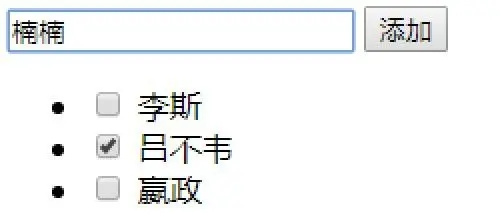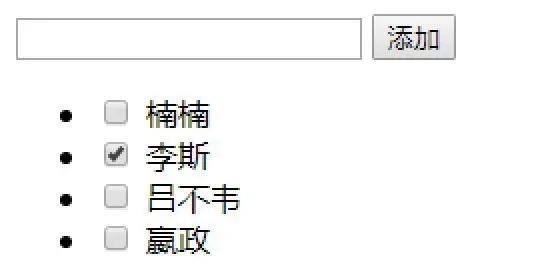123456789101112131415161718192021222324252627282930313233 `<``div` `id``=``"app"``>``  ``<``div``>``   ``<``input` `type``=``"text"` `v-model``=``"name"``>``   ``<``button` `@``click``=``"add"``>添加``  ````  ``<``ul``>``   ``<``li` `v-for``=``"(item, i) in list"` `:key``=``"item.id"``>``    ``<``input` `type``=``"checkbox"``> {{item.name}}``   ````  ````<``script``>``  ``// 创建 Vue 实例，得到 ViewModel``  ``var vm = new Vue({``   ``el: '#app',``   ``data: {``    ``name: '',``    ``newId: 3,``    ``list: [``     ``{ id: 1, name: '李斯' },``     ``{ id: 2, name: '吕不韦' },``     ``{ id: 3, name: '嬴政' }``    ``]``   ``},``   ``methods: {``    ``add() {``     ``//注意这里是unshift``     ``this.list.unshift({ id: ++this.newId, name: this.name })``     ``this.name = ''``    ``}``   ``}``  ``});`` ```` ```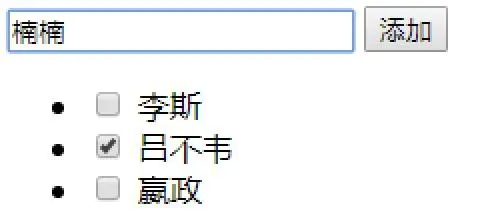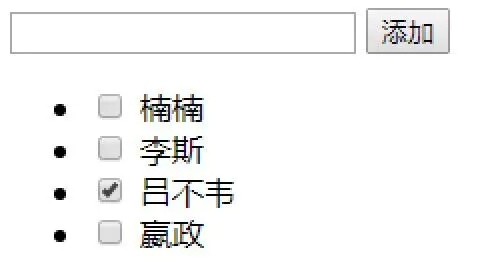vue和react的虚拟DOM的Diff算法大致相同，其核心是基于两个简单的假设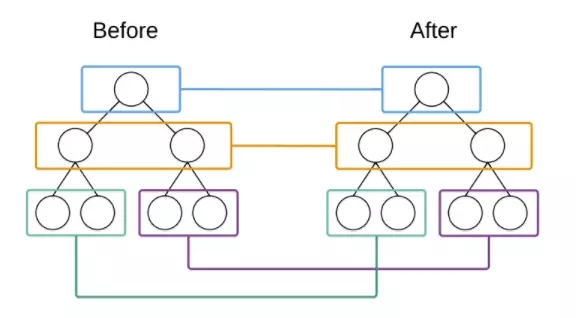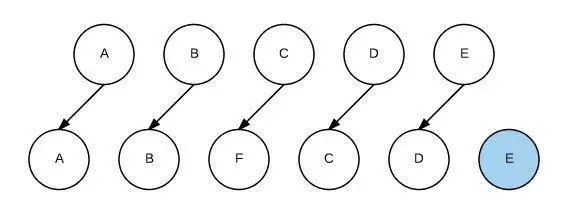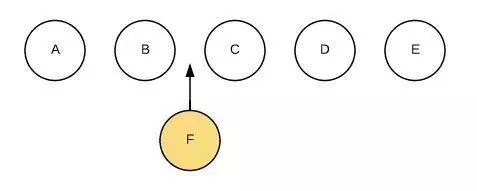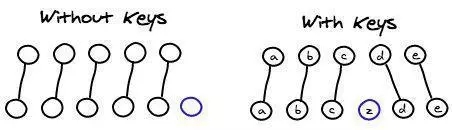vue中列表循环需加:key="唯一标识" 唯一标识可以是item里面id index等，因为vue组件高度复用增加Key可以标识组件的唯一性，为了更好地区别各个组件 key的作用主要是为了高效的更新虚拟DOM返回首页
 原文链接：https://www.jb51.net/article/178628.htm
 推荐资讯
 相关文章
 无相关信息
 栏目更新
 栏目热门